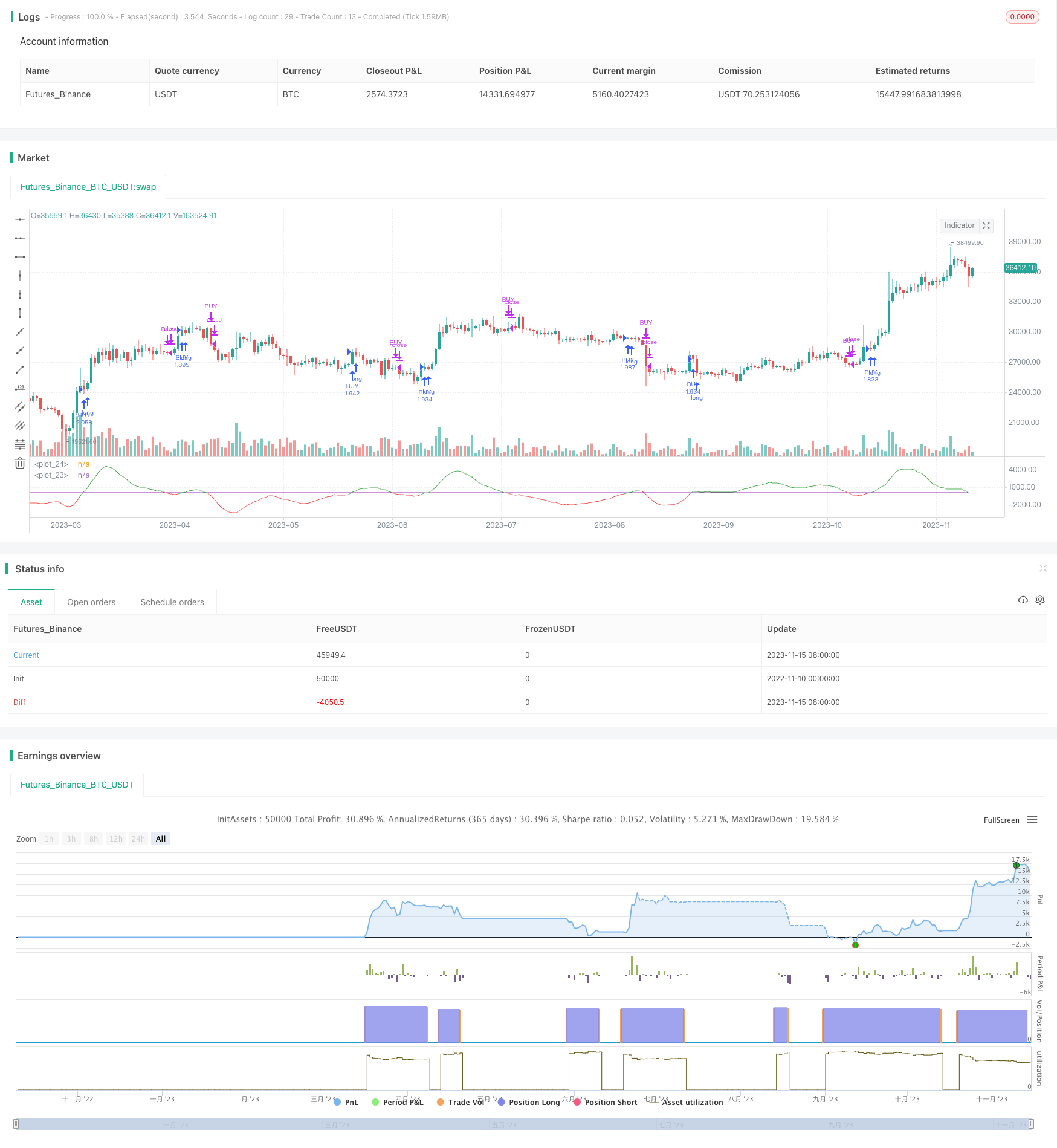# Bilinear Regression Trend Following Strategy

Author: ChaoZhang, Date: 2023-11-17 16:51:33
Tags:### Overview

The Bilinear Regression Trend Following strategy uses the difference between fast and slow linear regression to determine price trends and uses it as entry signals. It goes long when the fast linear regression crosses above the threshold and exits when it crosses below. It also uses EMA as a filter to only enter when price is above EMA.

### Strategy Logic

The strategy first calculates two linear regression lines with different periods, one fast with shorter period and one slow with longer period. Then it calculates the difference between the two, when fast regression is above slow regression, the difference is positive, indicating an uptrend. When fast is below slow, the difference is negative, indicating a downtrend.

The strategy enters long when the difference line crosses above the threshold and exits when it crosses below. It also requires price to be above 200-period EMA to filter out non-trending moves.

1. Dual linear regression can capture price trends well.

2. EMA filter eliminates some false signals from non-trending moves.

3. Simple and clear logic, easy to understand and implement.

### Risk Analysis

1. Improper LR periods may generate excessive noise.

2. EMA filter may miss opportunities in strong trends.

3. Prone to whipsaws and losses in ranging markets.

Solutions:

1. Optimize LR periods to reduce noise.

2. Dynamically adjust EMA period based on market conditions.

3. Add stop loss to control losses.

### Optimization

The strategy can be optimized in the following aspects:

1. Optimize fast and slow LR periods to find best combination.

2. Try other filters like Bollinger Bands, KDJ instead of EMA.

3. Add dynamic stop loss to control risks.

4. Combine with stock picking to select trending stocks.

5. Develop adaptive parameters based on market conditions.

### Summary

The Bilinear Regression strategy is simple and direct in capturing trends with dual linear regression and EMA filter. But it also has risks that need to be addressed through parameter optimization, stop loss, etc. When properly tuned, it can effectively trade trending markets.

```/*backtest
start: 2022-11-10 00:00:00
end: 2023-11-16 00:00:00
period: 1d
basePeriod: 1h
exchanges: [{"eid":"Futures_Binance","currency":"BTC_USDT"}]
*/

// This source code is subject to the terms of the Mozilla Public License 2.0 at https://mozilla.org/MPL/2.0/

//@version=4
strategy("Linear trend", overlay=false, default_qty_type=strategy.percent_of_equity, default_qty_value=100, commission_type=strategy.commission.percent, commission_value=0.075, currency='USD')
startP = timestamp(input(2017, "Start Year"), input(12, "Month"), input(17, "Day"), 0, 0)
end   = timestamp(input(9999, "End Year"),   input(1, "Month"),   input(1, "Day"),   0, 0)
_testPeriod() =>
iff(time >= startP and time <= end, true, false)

src = close
len1 = input(defval=13, minval=1, title="Fast LR")
len2 = input(defval=55, minval=1, title="Slow LR")

lag1 = input(0, title="Lag for fast")
lag2 = input(0, title="Lag for slow")

threshold  = input(0,step=0.5, title="Threshold")

fast_lr = linreg(src, len1, lag1)
slow_lr = linreg(src, len2, lag2)
lr = fast_lr - slow_lr
plot_fast = plot(lr, color = lr > 0 ? color.green : color.red)
plot(threshold, color=color.purple)

long_condition = crossover(lr, threshold) and close > ema(close, 200) and _testPeriod()